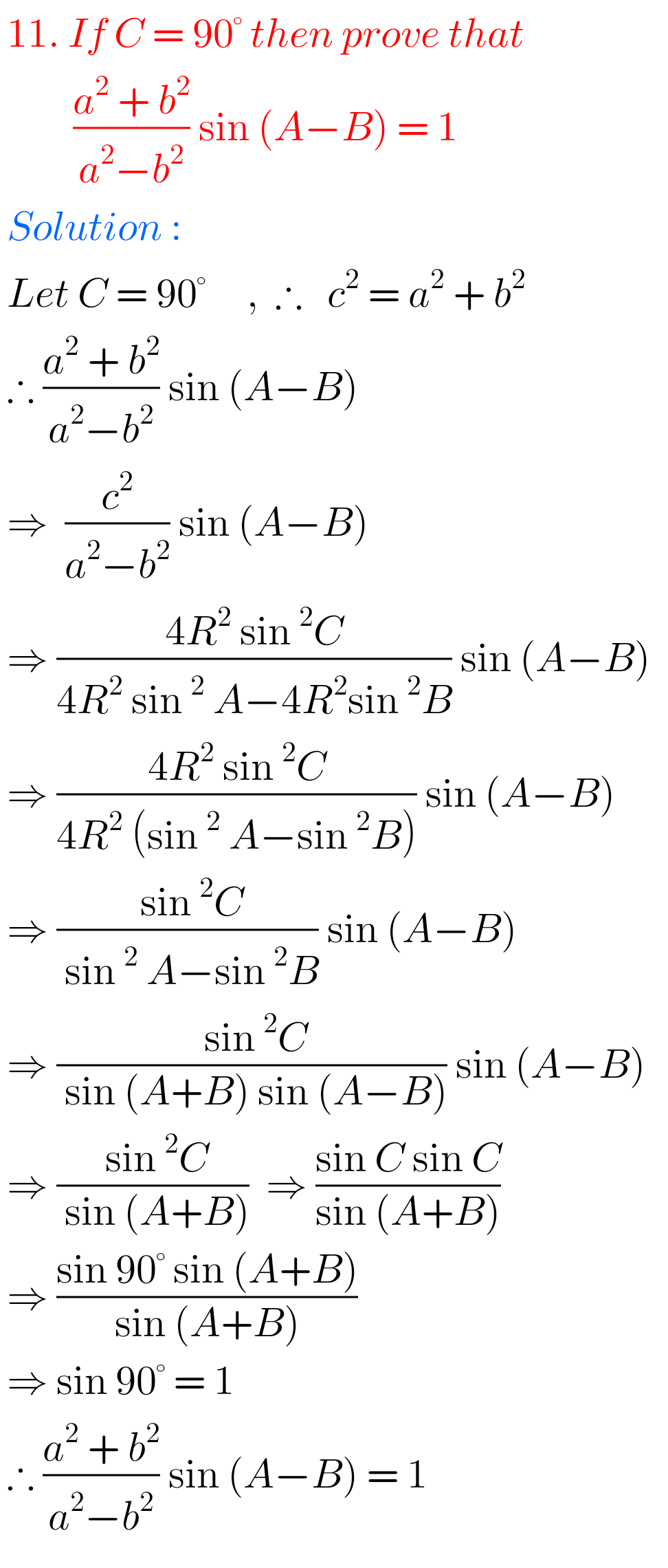## Intermediate Maths Solutions for Exercise 10(a) Properties of Triangles

Inter Maths Solutions for Exercise 10(a) Properties of Triangles Inter Maths 1A text book Exercise 10(a) Properties of Triangles solutions are given. These solutions are very easy to understand. Please study the text book lesson Properties of Triangles very well. Observe the example problems and solutions given in the text book. Observe the given solutions …